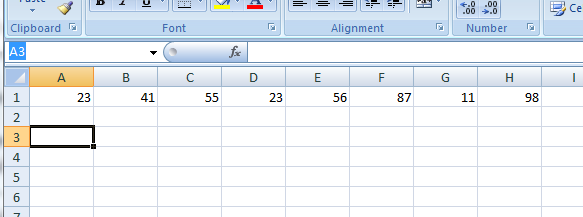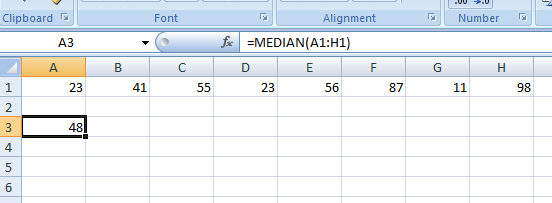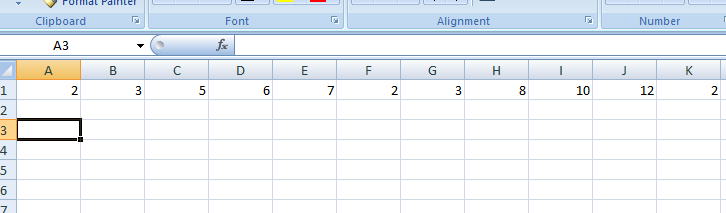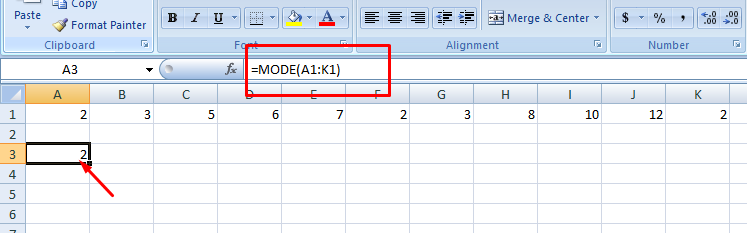# How to Find the Median in Maths?

Affiliate disclosure: In full transparency – some of the links on our website are affiliate links, if you use them to make a purchase we will earn a commission at no additional cost for you (none whatsoever!).

Mean, median and mode are the basic statistics functions which we all have learned in our childhood and no doubt they are extremely easy to find out.

But here is a catch! These statistics functions are actually very easy to calculate but only for those who loves maths or at least they are an average student of math.

Those with the love-hate relationship with maths, find these sums really difficult. But regardless of what kind of a student you have been in school, the computers have answers to every problem including how to find the median, mode and mean.Exactly, we can do such calculations on our computer systems very easily. The software we will be using for calculating statistics is Excel. Alongside to that, we will also tell you the real method i.e., the old school method of calculating the mean, mode, and median using a notebook and a pen/pencil.

Let’s get started with the tutorial.

## How to Find the Median:

There are two ways you can find the mean, median, and mode in maths. The first and very useful method is the stereotype method i.e., using the notebook which we have been doing from our childhood.

Although, there are 100% chances that you know how to find median and mode on the notebook, but you might not know our second method i.e., calculating median and mode on excel.

In this guide, you will get to learn how to find mode and median using both the old school method i.e., using copy/pen and using excel.

### How to Calculate Median:The median is the exact middle number of a set of numbers. Finding out the median of numbers is easy when you have the odd number of total numbers in a set as compared to the even numbers.

The old school method of finding out the Median is as follows.

Step 1. Arrange all the numbers in ascending or descending order. Let’s understand all this by taking an example. Suppose we have a series of the following numbers.

3, 5, 8, 7, 1, 4, 13, 14, 19, 2, 7, 12, 11

The total number of numbers in the set is 13, which is an odd number. Also, the data in the above set is not arranged in any particular order. But in order to find out the median, we need to arrange them in ascending or descending order. We are arranging them in ascending order.

1, 2, 3 ,4, 5, 7, 7, 8, 11, 12, 13, 14, 19

In the odd numbers, finding out the middle value is easy. You can easily judge the middle value in total of 13 is the 7th positioned value. In our case, it’s 7. So the median of the above set of data is 7.

But if we had the even numbers in the series, finding out the Median wouldn’t have been that easy. Suppose we have the following data to find the median of. The series is already arranged in ascending order. But you will have to arrange it if it’s not already arranged in your set of data.

So the series looks like,

1, 2, 3 ,4, 5, 7, 7, 8, 11, 12, 13, 14

Since we have even numbers in the above series, we will have to find out the average of the middle two numbers. In the above series, the two middle numbers are 7 and 7.

The average of these two numbers is (7+7)/2 i.e. 7. So the median of this even numbers of series is 7.

This is the old school method of finding out median. The second and the easiest method to finding out median is in Excel. It will hardly take just a few seconds of yours to get you the results. No need to arrange them in ascending or descending order, just a formula, and BOOM! The result will be on screen.

The steps are like this,

• Open excel sheet on your computer with the series of the data we want to find out the median of. See the following screenshot to checking out the data we will be finding out the median.• We have 8 numbers in the set. Now click on the cell where you would like to see the result and put the formula =median(A1:H1) and press enter.
A1 should be the cell reference where the series is starting, and H1 should be the cell reference of the last cell in the series. See the below screenshot to find out the median of the above series.So that’s how we find out the median of the numbers in excel.

### How to Find the Mode:

The mode in a series of numbers is the most frequently occurring number. In other words, the mode in a set of numbers is the number which appears the most often.

Finding out Mode in Excel is just a few seconds job. Let’s find out how to do it. Suppose we have the following series of number to find out the mode of.

2, 3, 5, 6, 7, 2, 3, 8, 10, 12, 2

These numbers in the series look’s like the below image.Now we don’t need to arrange them either in ascending or descending order in excel, just put the formula =Mode(A1:K1). A1 and K1 should be the first and last cell reference in the series respectively. Once you are done putting the formula, just hit enter you’ll see the following result.So the most occurred number in the series is 2. But if we want to find the mode of the above set of numbers manually using the old school method, we had to arrange them in ascending or descending order first. Like this,

2, 2, 2, 3, 3, 5, 6, 7, 8, 10, 12

Now count the number of times each number appeared i.e. 2 appeared 3 times, 3-two times, and rest of the numbers appeared one time each so clearly the Mode of the series is 2. This method is really time taking and lengthy if we have a large number of series.

We have told you both the ways on how to find the median and mode. Both methods are easy to apply. The first one is good when you have a smaller range of data while the other method applicable to every situation regardless of the range of numbers.

But it’s obvious doing a calculation manually using a notebook, pen, and a calculator is a time taking while doing the same calculation on Excel is far easier and quick.

You can use any of the methods according to your choice!

In the case of any doubt, you can drop your comment below; we will reach out to you as soon as possible!##### Diksha

A graduate of the IIMC, Diksha enjoys testing out new tech products and services. Her goal is to help others make more informed purchases of tech products and services. In her spare time, she enjoys traveling, watching movies, and listening to music. An ardent reader loves to be surrounded by technology.